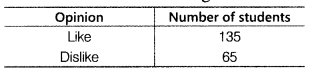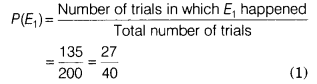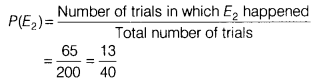# To know the opinion of the students about the subject Statistics, a survey of 200

To know the opinion of the students about the subject Statistics, a survey of 200 students was conducted. The data is recorded in the following tableFind the probability that a student chosen at random
(i) likes Statistics.
(ii) does not like Statistics.
If we double the above data, then the above probabilities will be change or not.

Given, total number of students = 200
∴ Total number of trials = 200
(i) Let E1, be the event of getting the number of students who like statistics.
∴ Number of trials in which E1 happened = 135
Now,(ii) Let E2 be the event of getting the number of students who dislike statistics.
∴ Number of trials in which E2 happened = 65
Now,The above probabilities will remain same, if we double the data.